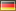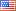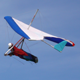OpenClonk Forum
Topic Development / Developer's Corner / Feature Request: Set Material of an Algorithm in Map ScriptBy FoalyDate 2019-03-14 19:58 Edited 2019-03-14 20:49Unless I'm mistaken, it is currently not possible to set a material of an Algorithm with map script.

(assume:
`var x = [0, 20, 20, 0];var y = [0, 0, 20, 20];`
)

For example, to draw a rectangle of earth, we can currently do this:
`map->Draw("Earth", { Algo = MAPALGO_Polygon, X = x, Y = y });`

However, it is not possible to draw multiple materials within one Draw call... Or is it?

The following code will draw two overlapping rectangles, one earth and one gold.
The overlapping part will remain sky, i. e. they are Xored (I chose this, because this would be more complicated to recreate with regular Draw, than operations like Or).
`map->Blit(  { Algo = MAPALGO_Xor, Op = [    { Algo = MAPALGO_And, Op = [{ Algo = MAPALGO_Polygon, X = x, Y = y }, CreateLayer("Earth")] },    { Algo = MAPALGO_And, Op = [{ Algo = MAPALGO_Offset, OffX = 10, OffY = 10, Op =      { Algo = MAPALGO_Polygon, X = x, Y = y }    }, CreateLayer("Gold")] }    ]});`

However, setting the material within a Blit call is inconvenient. It involves creating (or having several as local variables) a new dummy layer, just for the material.
I think it would be much nicer to have it this way:
`map->Blit(  { Algo = MAPALGO_Xor, Op = [    { Material = "Earth", Algo = MAPALGO_Polygon, X = x, Y = y },    { Material = "Gold", Algo = MAPALGO_Offset, OffX = 10, OffY = 10, Op =      { Algo = MAPALGO_Polygon, X = x, Y = y }    }  ]});`

Then, we wouldn't need temporary layers.

tl;dr

I want to add the extra property Material to Algorithms.
If it is set, then the algorithm will set that material.

Oh, and I forgot to mention:
If this would be an "accepted" feature, I could also try to implement this myself.By Sven2Date 2019-03-15 02:01 Edited 2019-03-15 02:06I don't have anything against such a feature (e.g. having a callback function to determine the material based on x/y?).

However, wouldn't it be easier if you just iterate over all pixels and set them individually? I.e., you use the existing Draw functions to create a dummy binary layer. Then you iterate over all the pixels of the layer that are set and use SetPixel to change them to whatever material you need.By FoalyDate 2019-03-15 07:13No, I didn't mean to have a callback function algorithm that is called for every pixel.

I just want to be able to set a material directly for the existing generator algorithms.By Luchs Date 2019-03-15 09:59I'm a bit worried about API symmetry here. With your proposal, what would the following do? Would it draw Earth or Gold?

`map->Draw("Earth", { Algo = MAPALGO_Polygon, X = x, Y = y, Material = "Gold" });`

The inconvenience of setting the material could be easily solved with a function:

`func AlgoMat(string mat, proplist algo){  return { Algo = MAPALGO_And, Op = [algo, CreateLayer(mat)] };}`

With that, your example would look like this:

`map->Blit(  { Algo = MAPALGO_Xor, Op = [    AlgoMat("Earth", { Algo = MAPALGO_Polygon, X = x, Y = y }),    AlgoMat("Gold", { Algo = MAPALGO_Offset, OffX = 10, OffY = 10, Op =      { Algo = MAPALGO_Polygon, X = x, Y = y }    })  ]});`

...which isn't more to type than what you propose.

>Then, we wouldn't need temporary layers.

You also worried about performance on IRC. I doubt it's much of an issue here. If it was, we could also optimize for this case, for example by having a special representation for completely-filled layers that does not allocate the pixels.By FoalyDate 2019-03-15 10:16> I'm a bit worried about API symmetry here. With your proposal, what would the following do? Would it draw Earth or Gold?
> `map->Draw("Earth", { Algo = MAPALGO_Polygon, X = x, Y = y, Material = "Gold" });`

It would draw it as Earth, matching the existing behavior. (i. e. the same as with setting the material via And and CreateLayer.)By Luchs Date 2019-03-15 14:34You're right, I looked at the code now. The material is an extra algorithm parameter that only layers set and that is ignored when using `Draw`.

So that changes my concerns a bit:

- With the extra Material property, we should just deprecate the Draw function to avoid the double material parameter. (FWIW, I think the current API at least has the conceptual distinction "Blit is for layers, Draw is for raw algorithms" that would be lost then.)

- An implementation of that Material property should not require keeping track of the material in the implementation of each algorithm, possibly by introducing an additional "Set Material" wrapper algorithm similar to the function I wrote in the parent comment. Any Material property would than desugar to that algorithm with the remaining proplist as child.By FoalyDate 2019-03-15 17:10I implemented the Material property.

Should I submit the patch?

Also I found a bug:
If the InitializeMap script crashes after having drawn sky, the engine crashes.
This is apparently, because `map->ConvertSkyToTransparent();` in C4MapScript.cpp:760 is only called for successful map creation.
It looks like it wouldn't hurt to always call it, which fixes the crash.By LuchsDate 2019-03-15 18:03>Should I submit the patch?

Yes, please do a pull request.
Topic Development / Developer's Corner / Feature Request: Set Material of an Algorithm in Map Script